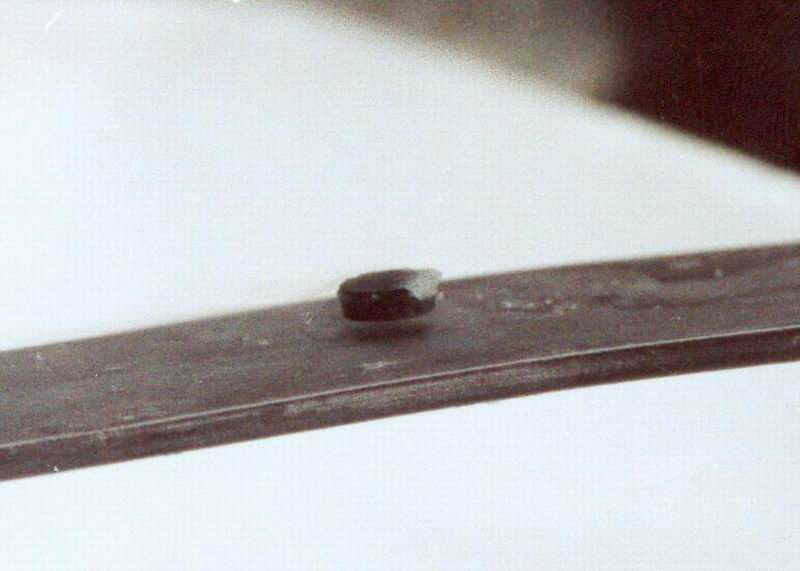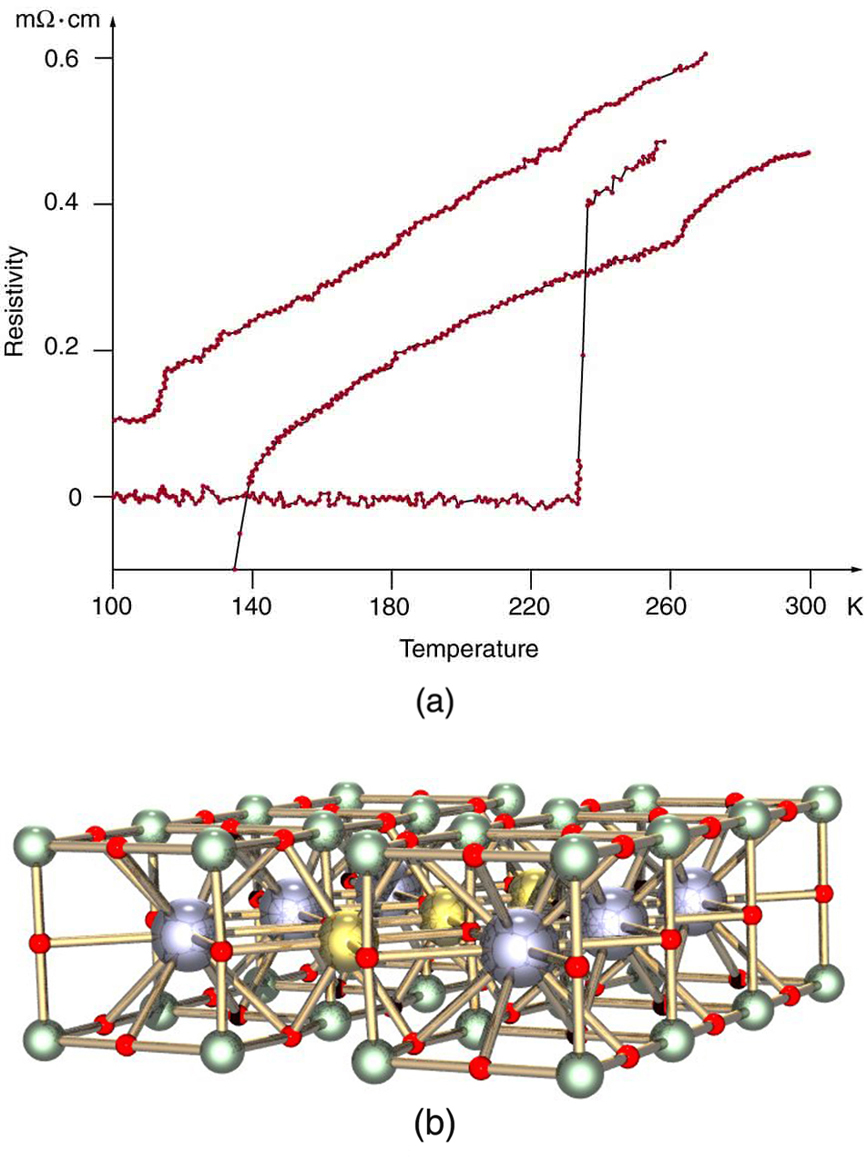# 34.6 High-temperature superconductors  (Page 2/4)

 Page 2 / 4One characteristic of a superconductor is that it excludes magnetic flux and, thus, repels other magnets. The small magnet levitated above a high-temperature superconductor, which is cooled by liquid nitrogen, gives evidence that the material is superconducting. When the material warms and becomes conducting, magnetic flux can penetrate it, and the magnet will rest upon it. (credit: Saperaud)

The search is on for even higher ${T}_{c}$ superconductors, many of complex and exotic copper oxide ceramics, sometimes including strontium, mercury, or yttrium as well as barium, calcium, and other elements. Room temperature (about 293 K) would be ideal, but any temperature close to room temperature is relatively cheap to produce and maintain. There are persistent reports of ${T}_{c}$ s over 200 K and some in the vicinity of 270 K. Unfortunately, these observations are not routinely reproducible, with samples losing their superconducting nature once heated and recooled (cycled) a few times (see [link] .) They are now called USOs or unidentified superconducting objects, out of frustration and the refusal of some samples to show high ${T}_{c}$ even though produced in the same manner as others. Reproducibility is crucial to discovery, and researchers are justifiably reluctant to claim the breakthrough they all seek. Time will tell whether USOs are real or an experimental quirk.

The theory of ordinary superconductors is difficult, involving quantum effects for widely separated electrons traveling through a material. Electrons couple in a manner that allows them to get through the material without losing energy to it, making it a superconductor. High- ${T}_{c}$ superconductors are more difficult to understand theoretically, but theorists seem to be closing in on a workable theory. The difficulty of understanding how electrons can sneak through materials without losing energy in collisions is even greater at higher temperatures, where vibrating atoms should get in the way. Discoverers of high ${T}_{c}$ may feel something analogous to what a politician once said upon an unexpected election victory—“I wonder what we did right?”(a) This graph, adapted from an article in Physics Today , shows the behavior of a single sample of a high-temperature superconductor in three different trials. In one case the sample exhibited a T c size 12{T rSub { size 8{c} } } {} of about 230 K, whereas in the others it did not become superconducting at all. The lack of reproducibility is typical of forefront experiments and prohibits definitive conclusions. (b) This colorful diagram shows the complex but systematic nature of the lattice structure of a high-temperature superconducting ceramic. (credit: en:Cadmium, Wikimedia Commons)

## Section summary

• High-temperature superconductors are materials that become superconducting at temperatures well above a few kelvin.
• The critical temperature ${T}_{c}$ is the temperature below which a material is superconducting.
• Some high-temperature superconductors have verified ${T}_{c}$ s above 125 K, and there are reports of ${T}_{c}$ s as high as 250 K.

## Conceptual questions

What is critical temperature ${T}_{c}$ ? Do all materials have a critical temperature? Explain why or why not.

Explain how good thermal contact with liquid nitrogen can keep objects at a temperature of 77 K (liquid nitrogen’s boiling point at atmospheric pressure).

Not only is liquid nitrogen a cheaper coolant than liquid helium, its boiling point is higher (77 K vs. 4.2 K). How does higher temperature help lower the cost of cooling a material? Explain in terms of the rate of heat transfer being related to the temperature difference between the sample and its surroundings.

## Problem exercises

A section of superconducting wire carries a current of 100 A and requires 1.00 L of liquid nitrogen per hour to keep it below its critical temperature. For it to be economically advantageous to use a superconducting wire, the cost of cooling the wire must be less than the cost of energy lost to heat in the wire. Assume that the cost of liquid nitrogen is $0.30 per liter, and that electric energy costs$0.10 per kW·h. What is the resistance of a normal wire that costs as much in wasted electric energy as the cost of liquid nitrogen for the superconductor?

$\text{0.30 Ω}$

what is physics
what are the basic of physics
faith
tree physical properties of heat
tree is a type of organism that grows very tall and have a wood trunk and branches with leaves... how is that related to heat? what did you smoke man?
what are the uses of dimensional analysis
Dimensional Analysis. The study of relationships between physical quantities with the help of their dimensions and units of measurements is called dimensional analysis. We use dimensional analysis in order to convert a unit from one form to another.
Emmanuel
meaning of OE and making of the subscript nc
Negash
kinetic functional force
what is a principal wave?
A wave the movement of particles on rest position transferring energy from one place to another
Gabche
not wave. i need to know principal wave or waves.
Haider
principle wave is a superposition of wave when two or more waves meet at a point , whose amplitude is the algebraic sum of the amplitude of the waves
kindly define principal wave not principle wave (principle of super position) if u can understand my question
Haider
what is a model?
hi
Muhanned
why are electros emitted only when the frequency of the incident radiation is greater than a certain value
b/c u have to know that for emission of electron need specific amount of energy which are gain by electron for emission . if incident rays have that amount of energy electron can be emitted, otherwise no way.
Nazir
Nazir
what is ohm's law
states that electric current in a given metallic conductor is directly proportional to the potential difference applied between its end, provided that the temperature of the conductor and other physical factors such as length and cross-sectional area remains constant. mathematically V=IR
ANIEFIOK
hi
Gundala
A body travelling at a velocity of 30ms^-1 in a straight line is brought to rest by application of brakes. if it covers a distance of 100m during this period, find the retardation.
just use v^2-u^2=2as
Gundala
how often does electrolyte emits?
alhassan
just use +€^3.7°√π%-4¢•∆¥%
v^2-u^2=2as v=0,u=30,s=100 -30^2=2a*100 -900=200a a=-900/200 a=-4.5m/s^2
akinyemi
what's acceleration
The change in position of an object with respect to time
Mfizi
Acceleration is velocity all over time
Pamilerin
hi
Stephen
It's not It's the change of velocity relative to time
Laura
Velocity is the change of position relative to time
Laura
acceleration it is the rate of change in velocity with time
Stephen
acceleration is change in velocity per rate of time
Noara
what is ohm's law
Stephen
Ohm's law is related to resistance by which volatge is the multiplication of current and resistance ( U=RI)
Laura
acceleration is the rate of change. of displacement with time.
the rate of change of velocity is called acceleration
Asma
how i don understand
how do I access the Multiple Choice Questions? the button never works and the essay one doesn't either
How do you determine the magnitude of force
mass × acceleration OR Work done ÷ distance
SeemaByBy Jazzycazz JacksonBy Marion CabalfinBy Olivia D'AmbrogioBy Brenna FikeBy OpenStaxBy OpenStaxBy Robert MurphyBy Dan ArielyBy Vanessa SoledadBy Sandhills MLT